### Home > A2C > Chapter 12 > Lesson 12.5.2 > Problem12-211

12-211.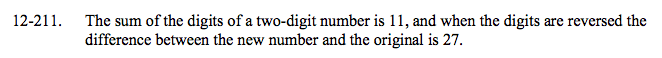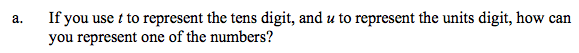10t + u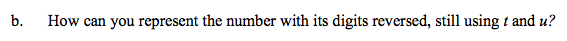See part (a). What effect does reversing the digits have?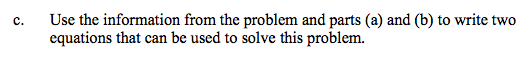How do you write an equation to find the sum of the digits?
How do you write an equation to find the difference of the numbers?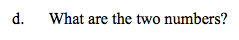74 and 47